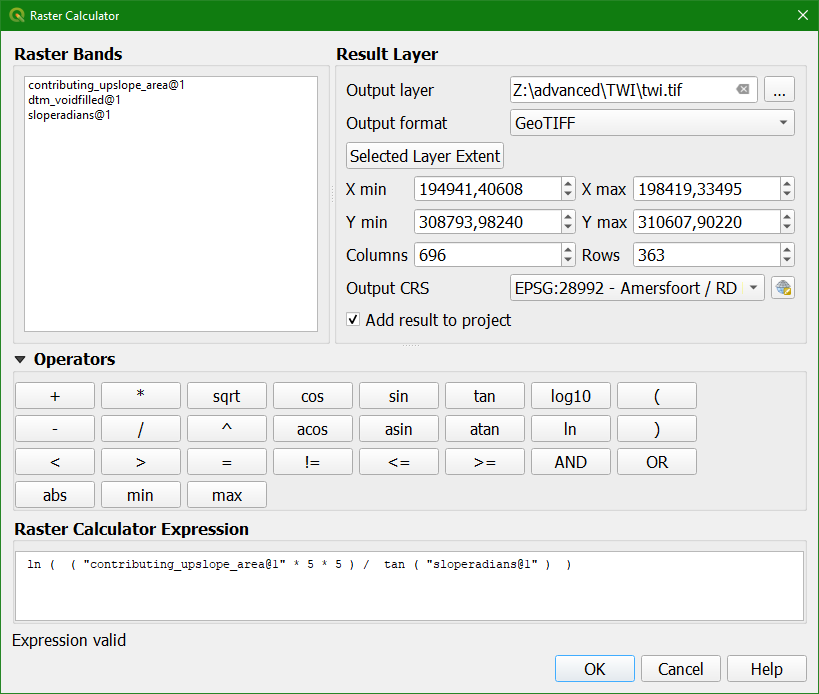## Tutorial: Calculate the Topographic Wetness Index from a DTM

### 5. Calculate TWI

Now we have the slope in radians and the upslope contributing area (in pixels) we can calculate the Topographic Wetness Index in the Raster Calculator.

1. Go to the Raster Calculator.

2. Write the following equation:

 ln ( ( "contributing_upslope_area@1" * 5 * 5 ) / tan ( "sloperadians@1" ) )

Note that we multiply the contributing upslope area (amount of pixels) with the area of the pixel (5 m x 5 m).3. Save the result to twi.tif.

Note that if the Output CRS is unknown, you need to set it for the input layer.

4. Click OK.

5. Style the result with a red to yellow to blue colour ramp and use blending with the hillshade layer.